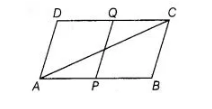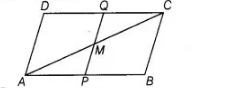# Points P and Q have been taken on`
Question:

Points P and Q have been taken on opposite sides AB and CD, respectively of a parallelogram ABCD such that AP = CQ. Show that AC and PQ bisect each other.Solution:

Given $A B C D$ is a parallelogram and $A P=C Q$

To show $A C$ and $P Q$ bisect each other.Proof In $\triangle A M P$ and $\Delta C M Q$,

$\angle M A P=\angle M C Q$   [alternate interior angles]

$A P=C Q$ [given]

and   $\angle A P M=\angle C Q M$    [alternate interior angles]

$\therefore$ $\triangle A M P \cong \triangle C M Q$ [by ASA congruence rule]

$\Rightarrow$ $A M=C M$ [by CPCT rule]

Hence, $A C$ and $P Q$ bisect each other.

Hence proved.